# How to collect like terms in algebra

• Objects are often represented in algebra by a single letter.

• Like terms share the same letter(s) and power(s), eg 𝑥 or 𝑥²

• Algebraic expressions can be collected together if they are like terms. This is done by adding or subtracting.

## Have a go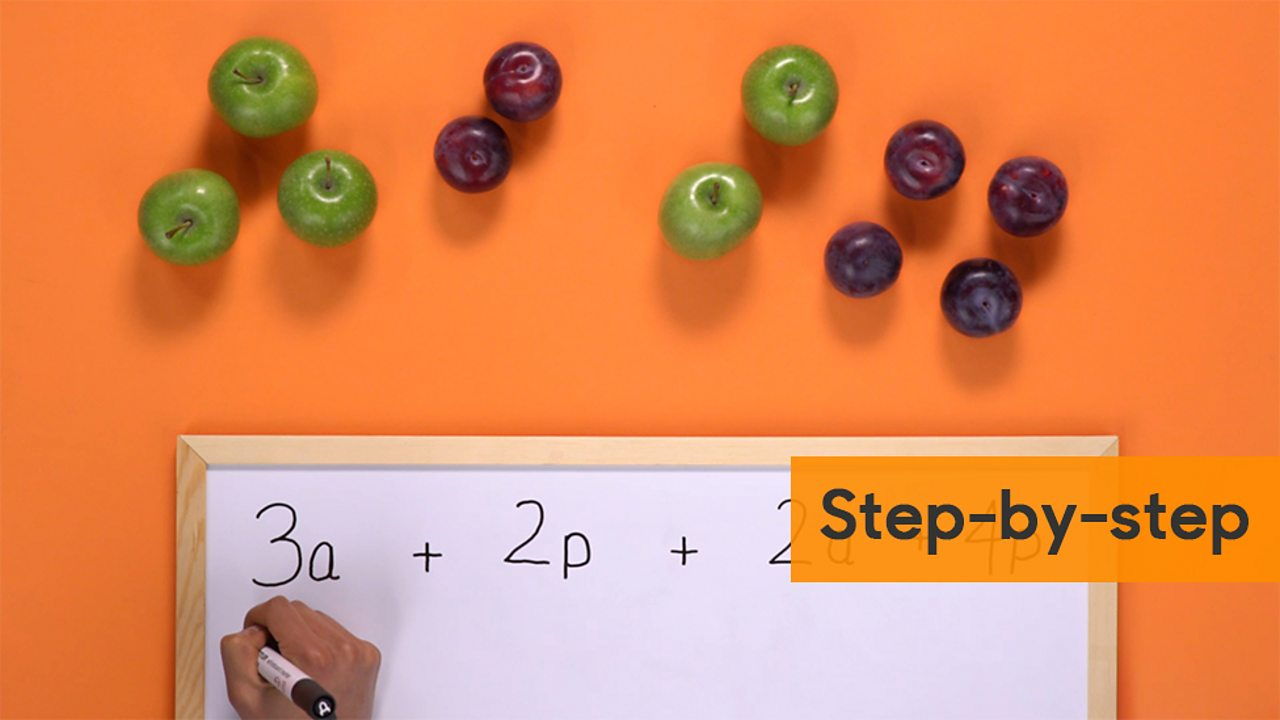Click to see a step-by-step slideshow.1 of 5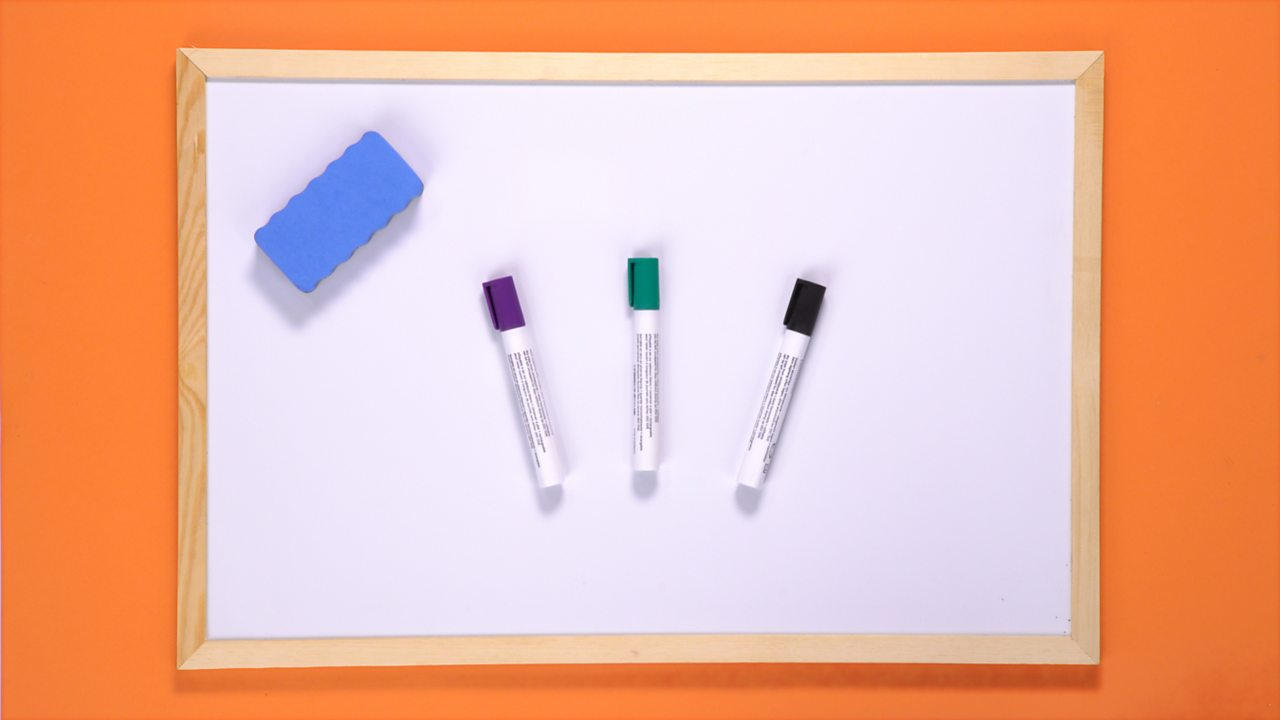YOU WILL NEED: A whiteboard, eraser and coloured pens.2 of 5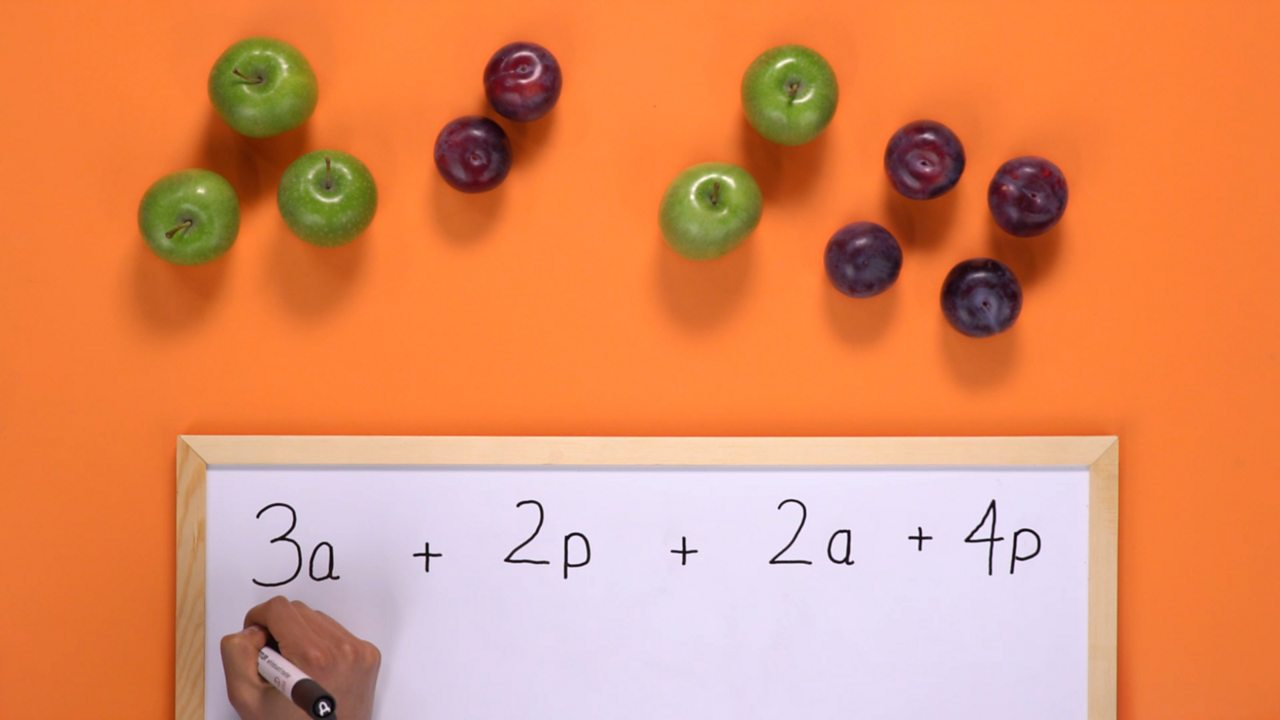STEP 1: Write out the equation using a and p to represent the apples and plums.3 of 5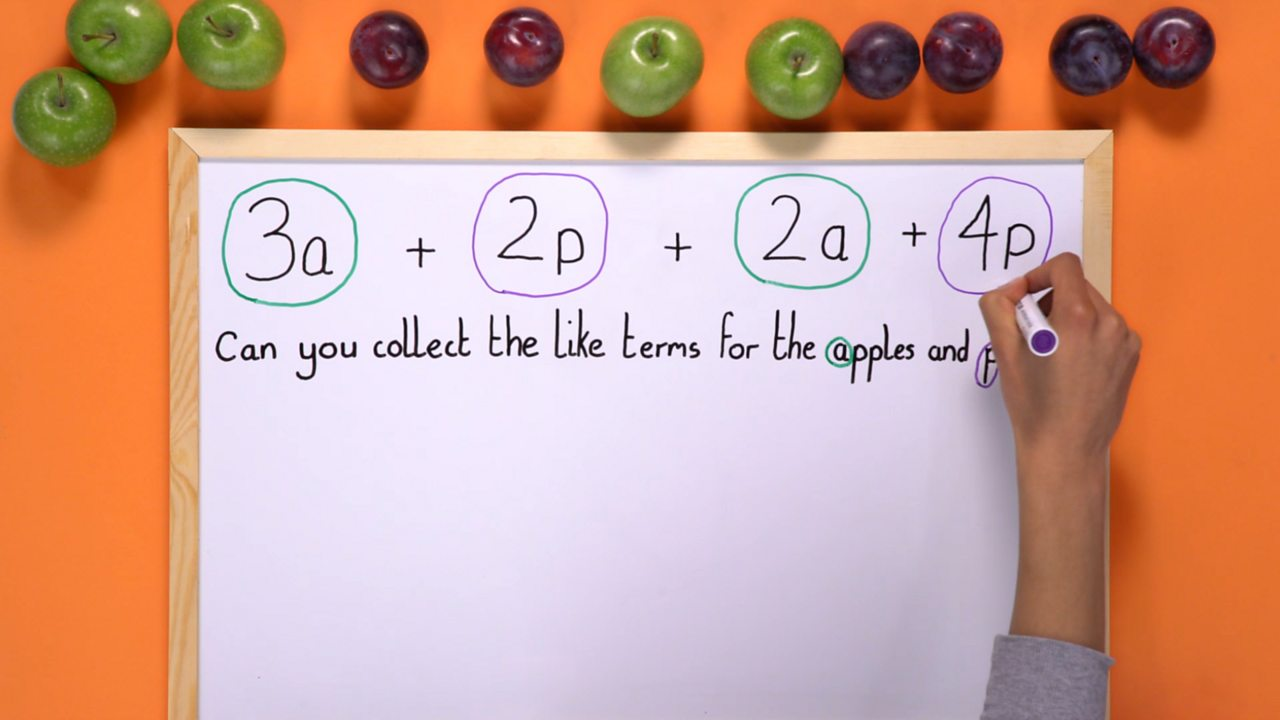STEP 2: Circle the 'like' terms in different colours.4 of 5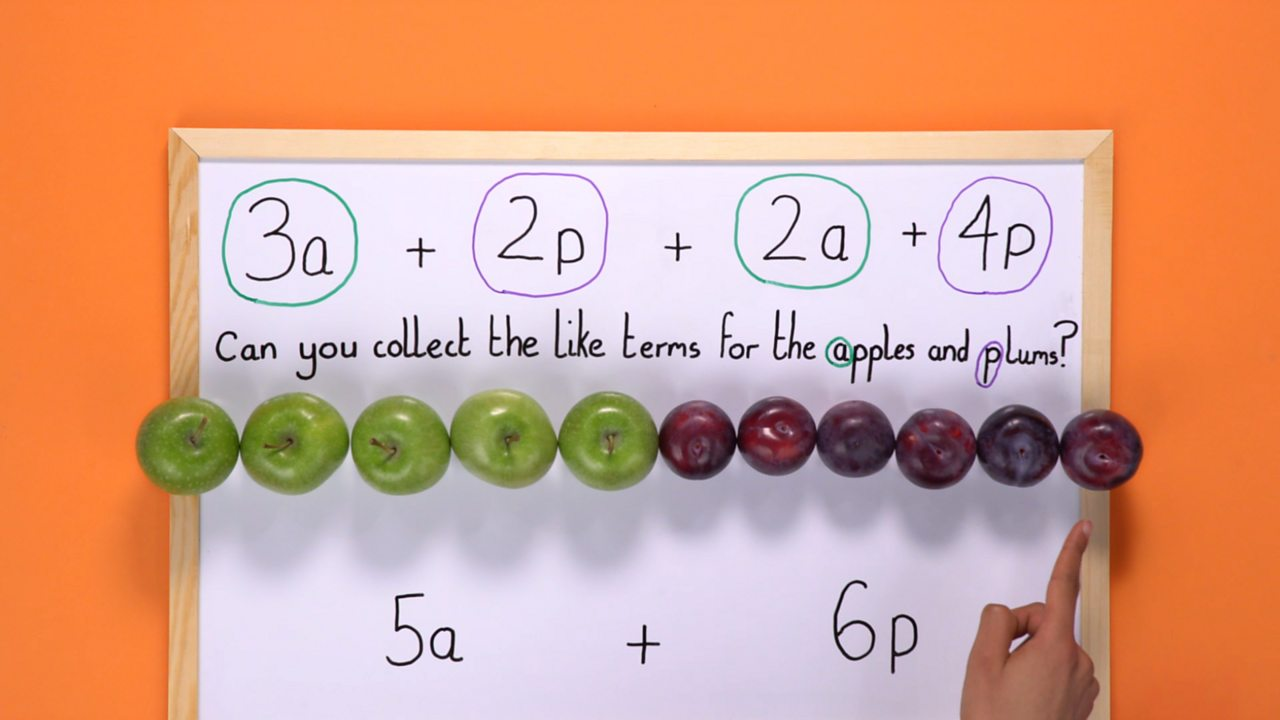STEP 3: Collect up the 'like' terms according to their colour.5 of 5

## How to represent a problem using terms

3 apples and 2 plums can be represented as:

3a + 2p

If we were to add a further 2 apples and 4 plums to this, we would represent this as:

3a + 2a + 2p + 4p

3a, 4p etc. are called terms. Numbers without letters, are also known as terms.

## What are 'like' terms?

Terms that contains the same letter(s) or power(s) are called like terms:

e.g. a, -21a and 550a are like terms. 13p, 440p and -2p are like terms. And 𝑥², 70𝑥² and -23𝑥² are all like terms, too.

## How to collect terms

### Example

3a + 2p + 2a + 4p

Firstly, re-write the equation so that the like terms are together:

3a + 2a + 2p + 4p

Finally use addition to collect them:

5a + 6p

## Collecting terms with different mathematical signs

When collecting terms, the sign at the front of the number (co-efficient) tells us if we need to add or subtract them.

### Example

3t - 2y + 10t - y - 12

Firstly, re-write the equation so that the like terms are together:

3t + 10t - 2y - y - 12

Finally, add or subtract where appropriate:

13t - 3y - 12

## Like terms quiz

Test your knowledge with this quiz.